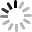# SVG 研究之路 (22) - 深入理解 SMIL Animation

## SVG 動畫屬性

SVG 的動畫具有以下幾個重要的也是共用的屬性，分別是：

如同 defs 定義，因為在 SVG 的使用上，往往會運用許多定義檔來構成圖形，因此我們可以運用 xlink:href 的方式，將動畫套用在具有某個 id 的圖形上，就不需要將動畫寫在圖形裏頭。

``````  <rect width="60" height="60" x="50" y="50" fill="#0c0" id="qq">
</rect>
<rect width="60" height="60" x="50" y="50" fill="none" stroke="#000">
</rect>
<animate dur="2s" attributeName="x" repeatCount="indefinite" to="100" xlink:href="#qq"/>
``````• attributeName

屬性的名稱，顧名思義就是當你設定了這個屬性，就會以這個屬性去進行動畫，下面的範例分別讓正方形水平和垂直移動。

``````  <rect width="60" height="60" x="50" y="50" fill="#c00">
<animate dur="2s" attributeName="x" repeatCount="indefinite" to="100"/>
</rect>
<rect width="60" height="60" x="50" y="50" fill="none" stroke="#000">
<animate dur="2s" attributeName="y" repeatCount="indefinite" to="100"/>
</rect>
``````• attributeType ( auto | CSS | XML )

屬性的類別，預設值為 auto，如果明確知道該屬性是 CSS 還是 XML ，就可以直接在這邊下定義，不過其實使用自動就好，因為會自動先去 CSS 的屬性找，找不到再去 XML 的屬性找，原則上來說應該都不會有問題。

## 動畫影格屬性

• calcMode ( discrete | linear | paced | spline )

指定動畫的進行速度模式，預設為 linear，也就是以同樣速度進行變化，如果遇到不支援的，則直接使用 discrete 的模式，直接跳到下一個狀態 ( from 直接跳到 to )，至於 paced ，則與 linear 相同，平均分配速度，不過就不能使用後面的 keyTimes 和 keySplines ，而 spline 就一定得用 keyTimes 和 keySplines 來配合運作。 ( 不清楚的話，繼續往下看，後面會有範例 )

• values

values 是一連串的值，中間使用「分號」隔開，也就是 from 到 to 之間的值，例如從 from="0" to="10" 進行了 10 秒，我們加上 value="0,2,10"，則 0-2 會跑五秒，2-10 會跑五秒。

``````  <rect width="60" height="60" x="50" y="50" fill="#f80">
<animate dur="2s" attributeName="x" values="50;60;100" repeatCount="indefinite"/>
</rect>
<rect width="60" height="60" x="50" y="50" fill="none" stroke="#000">
<animate dur="2s" attributeName="x" repeatCount="indefinite" to="100"/>
</rect>
``````• keyTimes

keyTimes 就是指動畫的時間，根據 W3C 的規範，若要使用 keyTimes，則必須遵守幾個原則：

• keyTimes 為 0-1 之間的浮點數。
• keytimes 後面的值一定要大於前面的值。
• 若和 values 做搭配，values 裡面有幾個值，設定 keyTimes 就要設定幾個值。
• 如果動畫只有 from 和 to，keyTimes 就只能設定兩個值。
• 如果使用了 linear 和 discrete，則 keyTimes 第一個值須為 0。
• 如果只有 from 和 to ，又設為無限重複 indefinite，則 keyTimes 將被忽略。
• keytimes 定義錯誤則會被忽略 。

下面這個是範例，可以看到時間不同的動畫移動：

``````      <rect width="60" height="60" x="50" y="50" fill="#c6c">
<animate dur="2s" attributeName="x" values="50;75;100" keyTimes="0;.9;1" repeatCount="indefinite"/>
</rect>
<rect width="60" height="60" x="50" y="50" fill="none" stroke="#000">
<animate dur="2s" attributeName="x" repeatCount="indefinite" to="100"/>
</rect>
``````• keySplines

keySplines 跟 CSS3 Cubic Bezier 其實超像的 ( 參考：CSS3 Cubic Bezier )，不過 keySplines 的彈性沒有 CSS3 Cubic Bezier 來得大，只能限定在 0-1 之間的範圍，而且必須與 keyTimes 一同使用，一個值裡面有四個數字，以分號隔開，比 keyTimes 少一個值，我們可由下圖看到藉由控制 keySplines，可以達到讓動畫加減速的效果。( 別忘記還要在 calcMode="spline" 的情形下使用)

``````  <rect width="60" height="60" x="50" y="50" fill="#0cc">
<animate dur="2s" attributeName="x" to="100" keyTimes="0;1" keySplines="0 .75 .25 1" repeatCount="indefinite" calcMode="spline"/>
</rect>
<rect width="60" height="60" x="50" y="50" fill="none" stroke="#000">
<animate dur="2s" attributeName="x" repeatCount="indefinite" to="100"/>
</rect>
``````• from

動畫起始時的屬性數值

• to

動畫結束時的屬性數值

• by

動畫相對於起始的屬性數值

## 動畫時間屬性

• begin

begin 可以說是動畫時間屬性裏頭最重要的了，上頭我們只提到了 begin 可以延遲時間，但其實 begin 裏頭卻有許多的學問：

• 時間設定

首先我們看到 begin 的時間單位，可以使用 h、min、s 與 ms 這四個單位，不過通常都是 s 和 ms 就足夠使用，因為要用 SVG 直接刻一個幾分鐘或幾小時的動畫，實在是...然而如果不給單位，預設的就是 s 秒數。

• id.end; id.begin

可以指定某個 id 的動畫結束之後再開始，還可以提早或延遲時間開始，或是指定某個動畫開始時就一起開始。

``````  <rect width="60" height="60" x="50" y="50" fill="#0cc">
<animate id="a1" dur="2s" attributeName="x" to="100"/>
<animate dur="2s" attributeName="y" to="100" begin="a1.end"/>
</rect>
<rect width="60" height="60" x="150" y="50" fill="#f80">
<animate id="a1" dur="2s" attributeName="x" to="200"/>
<animate dur="2s" attributeName="y" to="100" begin="a1.end+1s"/>
</rect>
<rect width="60" height="60" x="250" y="50" fill="#c00">
<animate id="a1" dur="2s" attributeName="x" to="300"/>
<animate dur="2s" attributeName="y" to="100" begin="a1.end-1s"/>
</rect>
``````• id.event;event

指定某個 id 的事件或是某個事件之後，就執行動畫，下面的範例，點選灰色矩形，就會執行動畫。

``````  <rect id="qq" width="120" height="30" x="50" y="10" fill="#aaa"/>
<rect width="60" height="60" x="50" y="50" fill="#0c0">
<animate dur="2s" attributeName="x" to="100" begin="qq.click"/>
</rect>
``````• id.repeat(整數)

某個 id 元素的動畫執行幾次之後就會接續執行。

``````  <rect width="60" height="30" x="50" y="50" fill="#c00">
<animate id="r1"   dur="1s" attributeName="width" to="120" repeatCount="indefinite" />
<animate dur="2s" attributeName="y" to="100" begin="r1.repeat(3)"/>
</rect>
``````• indefinite

可以直接使用超連結來控制動畫。

``````  <rect width="60" height="30" x="50" y="50" fill="#c00">
<animate id="a1" dur="2s" attributeName="y" to="100" begin="indefinite" repeatCount="indefinite"/>
</rect>
``````• dur

動畫的持續時間，除了時間單位，也可以設為 indefinite。

• end

動畫終止的時間。可指定多個值，以分號區隔，表示可在多個不同時間點終止，用法與 begin 相同。

• min / max

執行動畫最短和最長的時間，數值必須大於 0，min 其實沒有太直接的效果，而 max 倒是很容易可以看出來，當我們設定 max 為 10s，動畫將會在 10s 的時候停止。

``````  <rect width="60" height="60" x="50" y="50" fill="#c00">
<animate dur="4s" attributeName="x" repeatCount="indefinite" to="100" max="10s"/>
</rect>
<rect width="60" height="60" x="50" y="50" fill="none" stroke="#000">
<animate dur="4s" attributeName="x" repeatCount="indefinite" to="100"/>
</rect>
``````• restart (always | whenNotActive | never)

剛剛上面的範例有提到「可以使用超連結來控制動畫」，以及某個 id 點擊事件來觸發動畫，但使用的過程中會發現，每點一次動畫就跑一次，坦白說還真是有點討厭，這時候就必須使用 restart 的屬性，restart 總共有三個設定值，預設是 always，也就是每點一次就會執行一次，而 never 也很好理解就是永遠不會再觸發點擊的動作，另外一個設定就是 whenNotActive，這表示動畫正在執行的時候，點擊是沒有做用的。下面的範例設定動畫跑了三秒會停止，在動畫進行中，怎麼點擊按鈕都是沒有反應的。

``````  <rect width="30" height="30" x="50" y="50" fill="#c00">
<animate id="a1" dur="1s" attributeName="width" to="100" begin="indefinite" repeatCount="indefinite" restart="whenNotActive" max="3s"/>
</rect>
``````• repeatCount

動畫執行的次數，設定 indefinite 則是無限次循環。

• repeatDur

動畫執行的時間，設定 indefinite 則是無限長。

• fill ( freeze | remove )

這裡的 fill 不是填色的 fill，而是提供動畫結束時的一個屬性，屬性包含了 freeze 和 remove，remove 是預設值，也就是動畫結束之後，圖形返回原本的狀態，而 freeze 則是在動畫結束後，固定在結束的狀態。

``````  <rect width="60" height="60" x="50" y="50" fill="#c00">
<animate dur="2s" attributeName="x" to="100" fill="freeze"/>
</rect>
<rect width="60" height="60" x="50" y="50" fill="none" stroke="#000">
<animate dur="2s" attributeName="x" to="100" fill="remove"/>
</rect>
``````## 動畫添加與合併屬性

• additive ( replace | sum )

這是可以把動畫效果進行置換 replace 或相加 sum 的屬性，例如在 animateTransform 裏頭，type 會有 rotate、traslate、scale 和 skew 等設定，如果我們使用 replace，後面的動畫就會置換掉前面的動畫，使用 sum 就會將兩個動畫效果加在一起。下面的範例把位移、放大和旋轉三個效果合而為一，因為放大會改變位置，所以使用 translate 把位置調整回來。

``````  <rect width="60" height="60" x="50" y="50" fill="#069">
<animateTransform attributeName="transform" dur="2s" type="translate" from="50,50" to="-50,-50"  repeatCount="indefinite" additive="sum"/>
<animateTransform attributeName="transform" dur="2s" type="scale" from="1" to="2"  repeatCount="indefinite" additive="sum"/>
<animateTransform attributeName="transform" dur="2s" type="rotate" from="0,80,80" to="360,80,80" repeatCount="indefinite" additive="sum"/>
</rect>
``````• accumulate ( none | sum )

設定動畫結束後，是否從結束的地方接續動畫下去，none 是預設值，如果我們使用 sum，則會在第一段動畫結束的狀態繼續往下加，例如一開始縮放是 1>2，下一段就是2>4，下一段就是4>6，依此類推，不過使用者個屬性有規定，如果不是重複的動畫就不能使用。

``````  <rect width="10" height="10" x="0" y="0" fill="#069">
<animateTransform attributeName="transform" dur="2s" type="scale" from="1" to="2"  repeatCount="indefinite" accumulate="sum"/>
</rect>
<rect width="10" height="10" x="0" y="0" fill="#c00">
<animateTransform attributeName="transform" dur="2s" type="scale" from="1" to="2"  repeatCount="indefinite" accumulate="none"/>
</rect>
``````